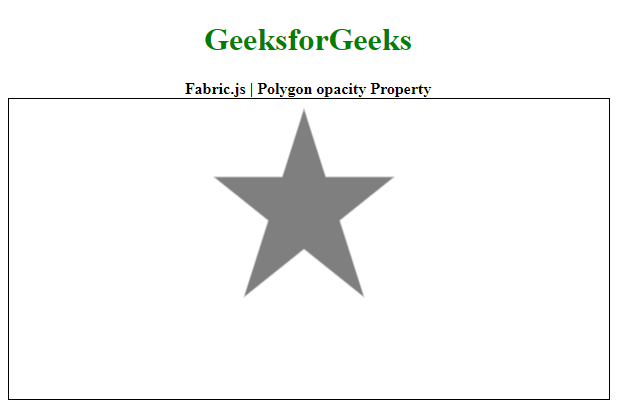Skip to content
Related Articles
Fabric.js Polygon opacity Property
• Last Updated : 31 Aug, 2020

In this article, we are going to see how to set the opacity of a canvas polygon using FabricJS. The canvas polygon means the polygon is movable and can be stretched according to requirement. Further, the polygon can be customized when it comes to initial stroke color, shape, fill color, or stroke width.

To make it possible we are going to use a JavaScript library called FabricJS. After importing the library, we will create a canvas block in the body tag that will contain the polygon. After this, we will initialize instances of Canvas and polygon provided by FabricJS and set the opacity of the polygon using opacity property and render the polygon on the Canvas as given in the example below.

Syntax:

```fabric.Polygon([
{ x: pixel, y: pixel },
{ x: pixel, y: pixel },
{ x: pixel, y: pixel },
{ x: pixel, y: pixel },
{ x: pixel, y: pixel }],
{
opacity: number
}
)
```

Parameters: This function accepts a single parameter as mentioned above and described below:

• opacity: It is a number that specifies the opacity of the canvas polygon.

Note: The dimension pixels are a must for creating a polygon.

Below example illustrates the Polygon opacity Property in JavaScript:

Example:

## HTML

 ```<``html``>`` ` `<``head``>``    ````    ``<``script` `src``=``"https://cdnjs.cloudflare.com/ajax/libs/fabric.js/3.6.2/fabric.min.js"``>``    `````` ` `<``body``>``    ``<``div` `style="text-align: center;``              ``width: 600px;">``        ``<``h1` `style``=``"color: green;"``>``            ``GeeksforGeeks``        ````        ``<``b``>``            ``Fabric.js | Polygon opacity Property``        ````    ```` ` `    ``<``canvas` `id``=``"canvas"` `width``=``"600"` `height``=``"300"` `        ``style``=``"border:1px solid #000000;"``>``    ````     ` `    ``<``script``>`` ` `        ``// Initiate a Canvas instance ``        ``var canvas = new fabric.Canvas("canvas");`` ` `        ``// Initiate a polygon instance ``        ``var polygon = new fabric.Polygon([``            ``{ x: 295, y: 10 },``            ``{ x: 235, y: 198 },``            ``{ x: 385, y: 78 },``            ``{ x: 205, y: 78 },``            ``{ x: 355, y: 198 }], {`` ` `            ``// Set the opacity of``            ``// the polygon``            ``opacity: 0.5`` ` `        ``});`` ` `        ``// Render the polygon in canvas ``        ``canvas.add(polygon); ``    `````` ` ``

Output:My Personal Notes arrow_drop_up